# Skewed Distribution

Published:

This lesson covers Introduction to Skewed Distribution.

Sources:

• https://www.statisticshowto.com/probability-and-statistics/skewed-distribution/
• http://jse.amstat.org/v13n2/vonhippel.html
• https://www.statisticshowto.com/skewness/

# What is a Skewed Distribution?

If one tail is longer than another, the distribution is skewed.

• aka Asymmetric or Asymmetrical distributions

Symmetry means:

• one half of the distribution is a mirror image of the other half
• The tails are exactly the same, e.g. normal distribution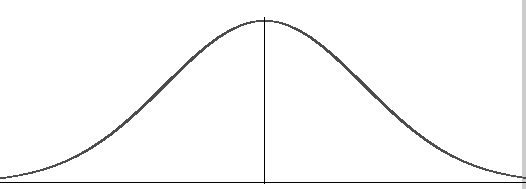## Left-Skewed

Long left tail

• aka negatively-skewed distributions
• long tail in the negative direction on the number line. The mean is also to the left of the peak.
• Mean is to the left of the peak. This is the main definition behind “skewness”, which is technically a measure of the distribution of values around the mean.
• In most cases, the mean is to the left of the median. This isn’t a reliable test for skewness though, as some distributions (i.e. many multimodal distributions) violate this rule. You should think of this as a “general idea” kind of rule, and not a set-in-stone one.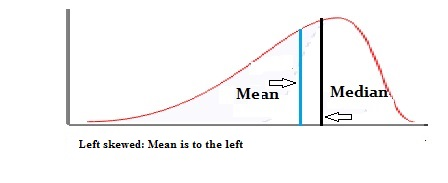• Box Plot (Left Skewed)

• Left Whisker is longer than right whisker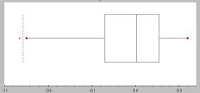• Histogram (Left Skewed)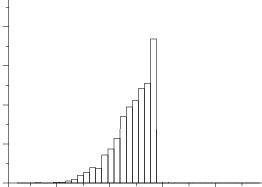## Right-Skewed

Long right tail

• aka positive-skew distributions
• long tail in the positive direction on the number line. The mean is also to the right of the peak.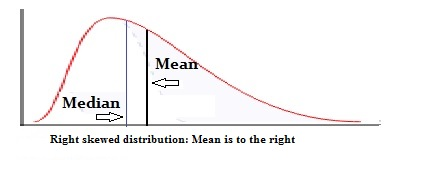• Histogram (Right Skewed)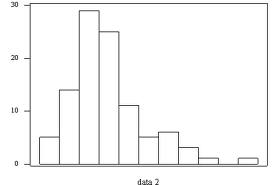• Box Plot (Right Skewed)

• Right whisker is longer than left whisker.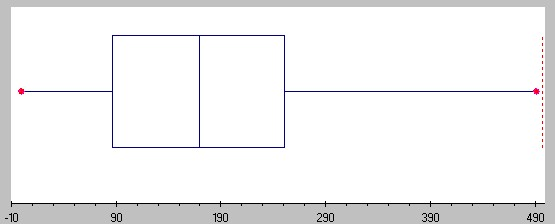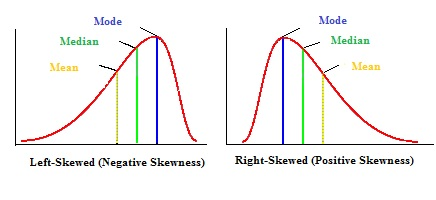• Example:
• Numbers: $1, 2, 3$
• Evenly spaced, with $2$ as the mean
• Adding a number to the far left: $-10,~ 1,~ 2,~ 3$
• Left skewed
• Adding a value to the far right: $1,~ 2,~ 3,~ 10$
• Right skewed

# Exception

• Distribution from a 2002 General Social Survey. Respondents stated how many people older than 18 lived in their household.
• Right-skewed graph, but the mean is clearly to the left of the median.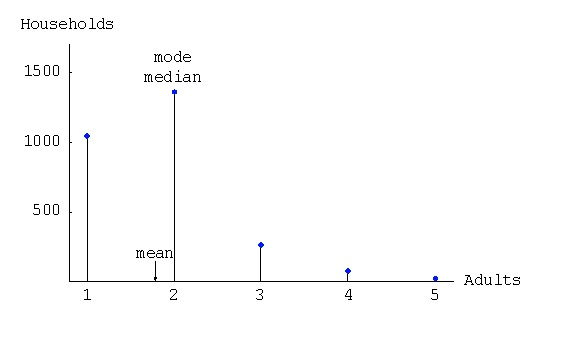# Compute Skewness

## Calculation

• Pearson Mode Skewness

• The mean, mode and median can be used to figure out if you have a positively or negatively skewed distribution.
• If the mean is greater than the mode, the distribution is positively skewed.
• If the mean is less than the mode, the distribution is negatively skewed.
• If the mean is greater than the median, the distribution is positively skewed.
• If the mean is less than the median, the distribution is negatively skewed.
• $Skew = \frac{Mean – Mode}{Standard~ Deviation}$
• Mode Skeweness
• Alternative Pearson Mode Skewness

• $Skew = 3 * \frac{Mean – Median}{Standard~ Deviation}$
• Median Skewness
• MS Excel

• SKEW function is used to calculate the skewness of the sample data
• Excel uses adjusted Fisher-Pearson standardized coefficient
• $G = \frac{n}{(n-1)(n-2)} \Sigma(\frac{x_i - \bar{x}}{s})^3$
• $s$ - STDEV.S in excel for Sample
• SKEW.P function is used to calculate the skewness of the population data
• $S_k = \frac{1}{n} \Sigma(\frac{x_i-\mu}{\sigma})^3$
• Data

• $X = {1,1,~~ 2,2,2,2,2,~~ 3,3,3,3,~~ 5,5,~~ 7, 8}$

• Plot Histogram
• Insert -> Histogram
• Right Click Data Area -> Format Data Series -> Bin Width 0.9
• Right Skewed
• $SKEW.P() \implies 1.09899799$
• $X = 1, 2,~~ 3,3,~~ 5,5,5,~~ 7,7,7,7,~~ 8,8,8,8,8$

• Plot Histogram

• Insert -> Histogram
• Right Click Data Area -> Format Data Series -> Bin Width 0.9
• Left Skewed
• $SKEW.P() \implies -0.704386$

Tags: